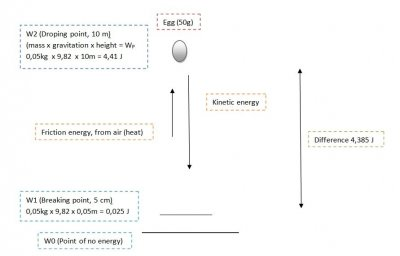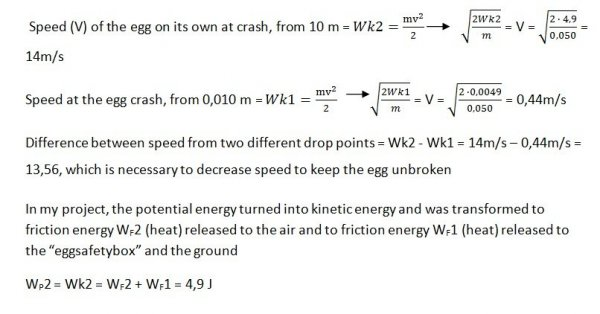The law of conservation of energy says"Energy cannot be made or disappear, only be transformed into different shapes."

To keep the eggshell unbroken in a fall from 10 m, I need to decrease the kinetic energy and the speed, otherwise it will break.

This is possible by transforming the kinetic energy into friction energy (heat) released to the air, with help from a parachute and by a shock absorber in a form of an “eggfallsafetybox”, where it also turns to friction energy (heat).

In my project I have succeeded to transform the potential energy to kinetic energy and finely to friction energy (heat). And in this whey keep the egg unbroken.

Formulas and calculations:

Weight of an standard egg = 0,050 kg (50g)

The height from where the egg is dropped = 10 m

Height of where the egg breaks = just above 0,010 m (tested)

Gravitation in Sweden = 9,82 m/s2Force (F) = mass x gravitation = 0,050kg x 9,82 m/s2 = 0,49 N

Potential energy WP = mgh (mass x gravitation x height) in Joule (J)

Potential energy at drop point, 10 m (WP2) = mgh, 0,050 kg x 9,82 x 10 m = 4,9 J

Potential energy at safe point, 0,010 m (WP1) = mgh, 0,050 kg x 9,82 x 0,010 m = 0,0049 J

Difference between drop point and safe point = WP2 - WP1 = 4,9 J - 0,0049 J = 4,8951 J, which is necessary to decrease kinetic energy to keep the egg unbroken

Kinetic energy =

Kinetic energy at crash point of egg on its own= Wk2 = Wp2 = 4,9 J